# RD Sharma Solutions For Class 7 Maths Chapter 20 Mensuration - I (Perimeter and Area of Rectilinear Figures) Exercise 20.3

RD Sharma Solutions for Class 7 Maths Exercise 20.3 of Chapter 20 Mensuration – I (Perimeter and Area of Rectilinear Figures) in PDF are available here. Learners can download the PDF from the provided links. RD Sharma Solutions for Class 7 provides answers to all the problems present in the textbook. This exercise deals with the areas of a parallelogram and a rhombus, formula to calculate base and altitude of the parallelogram. Solutions here are developed with utmost care to help students analyze the problems competently and hence, score good marks in their exams.

## Download the PDF of RD Sharma Solutions For Class 7 Maths Chapter 20 – Mensuration – I (Perimeter and Area of Rectilinear Figures) Exercise 20.3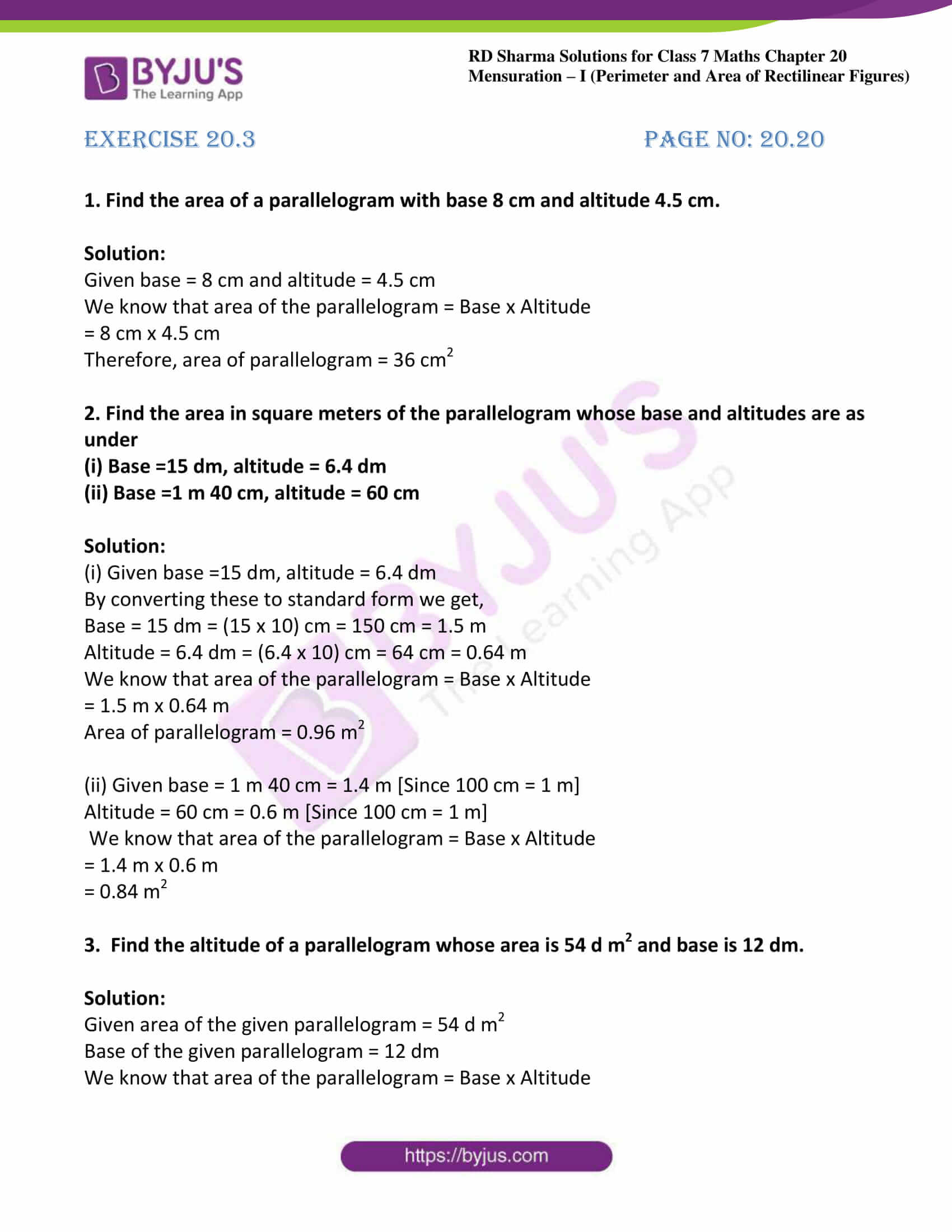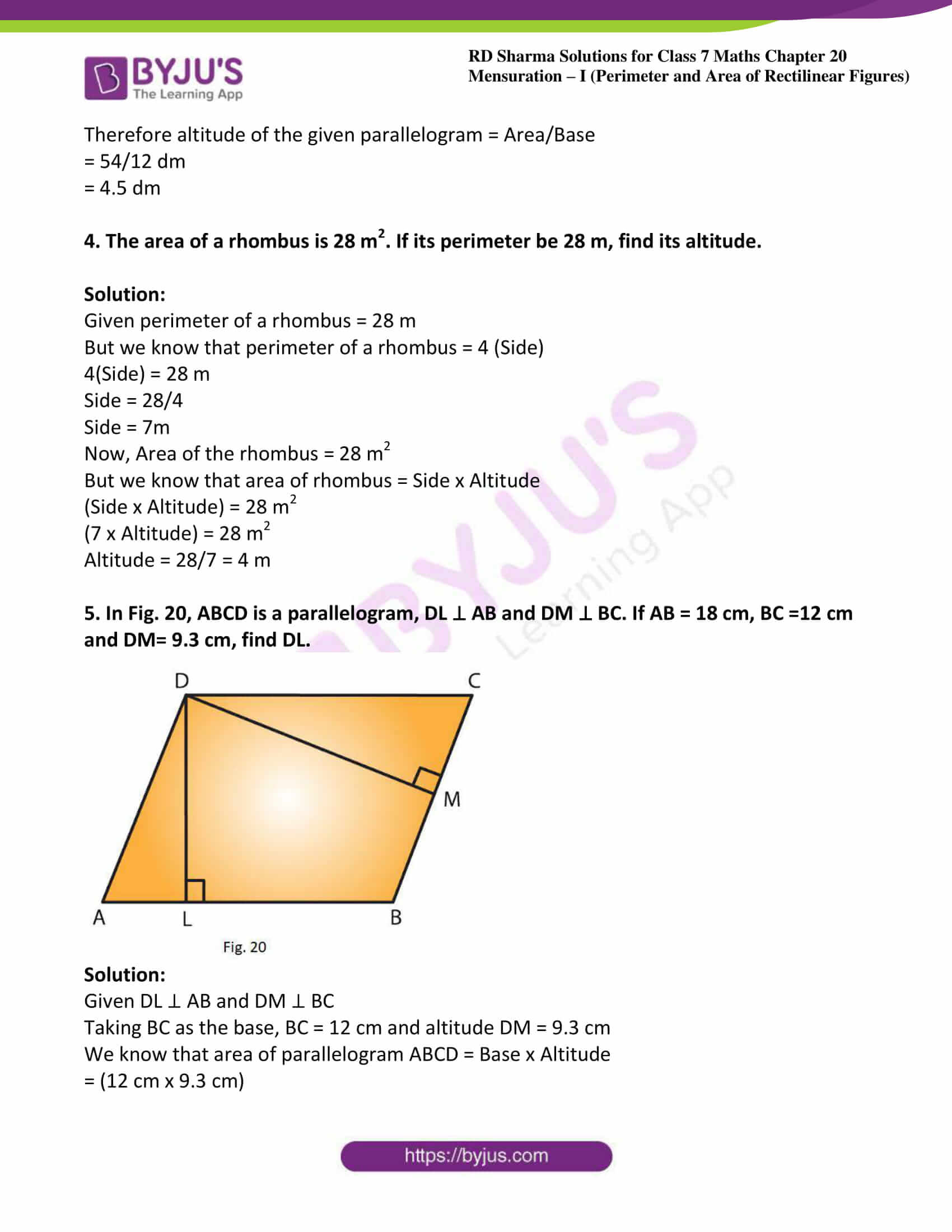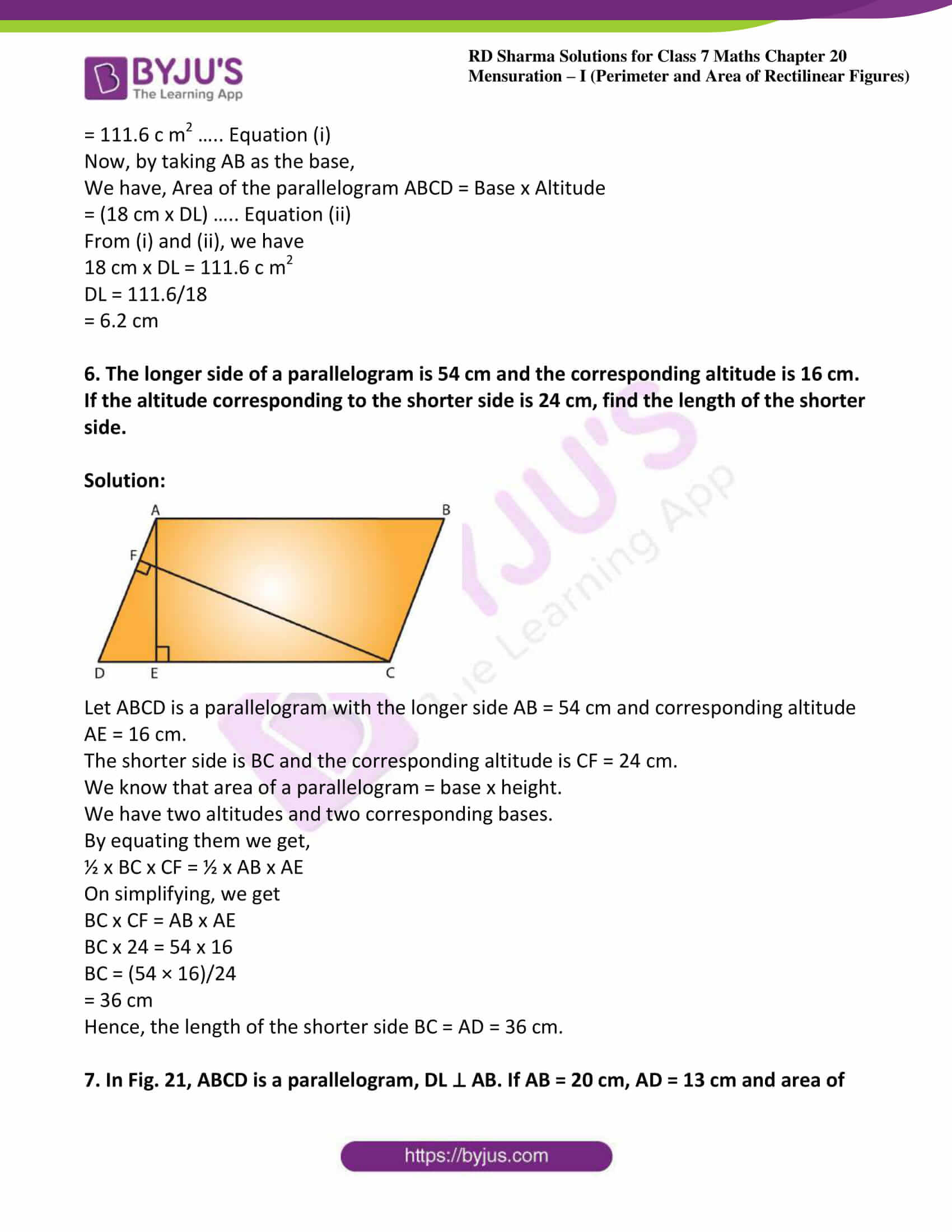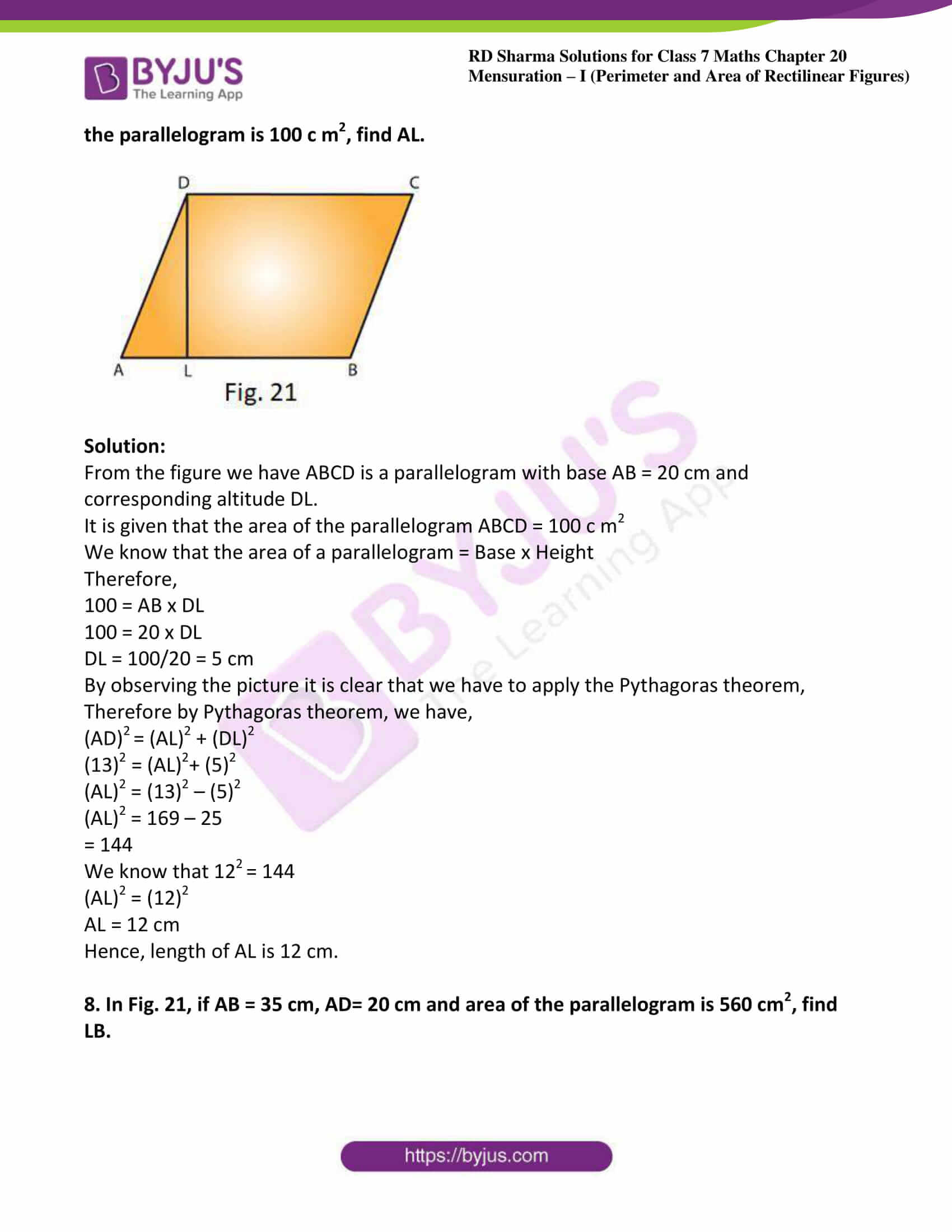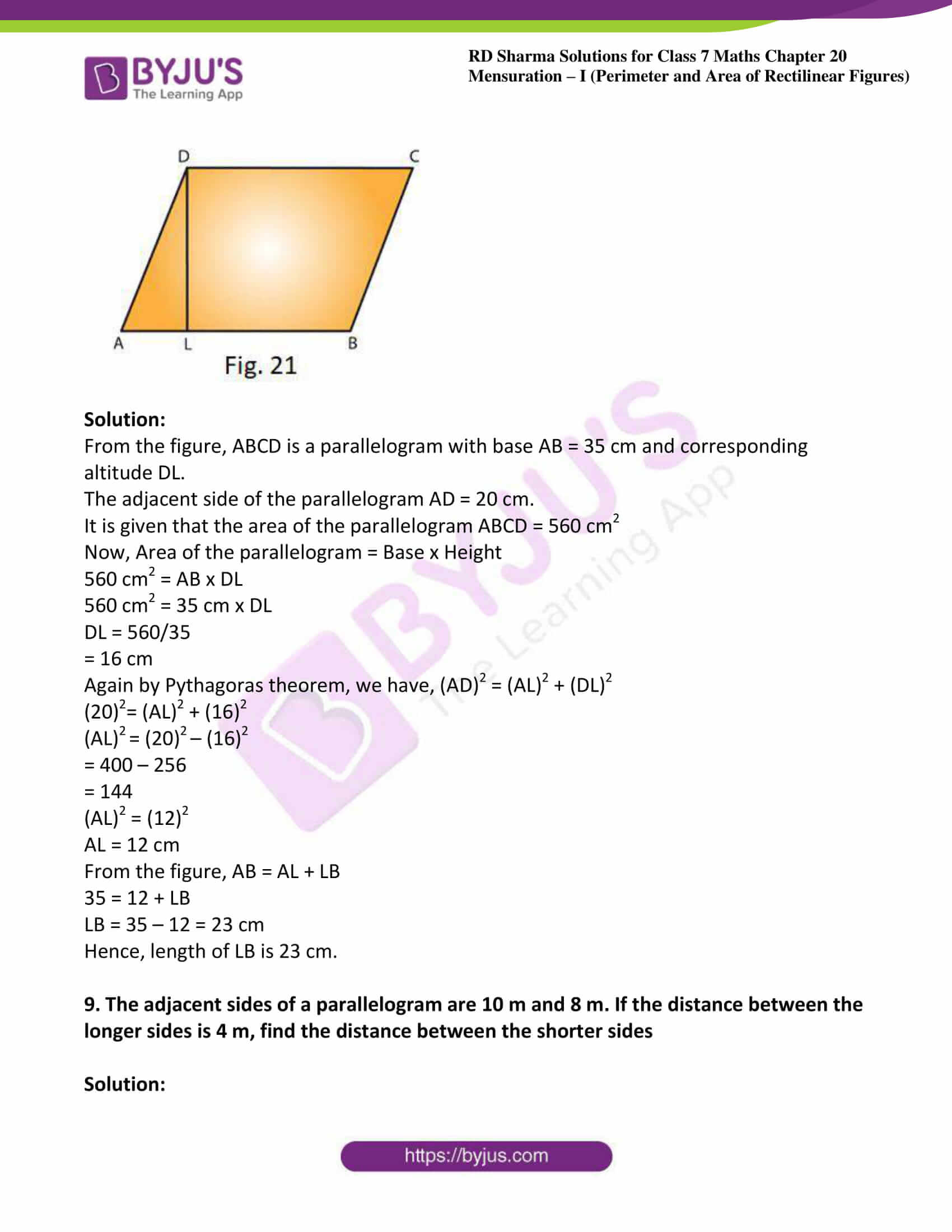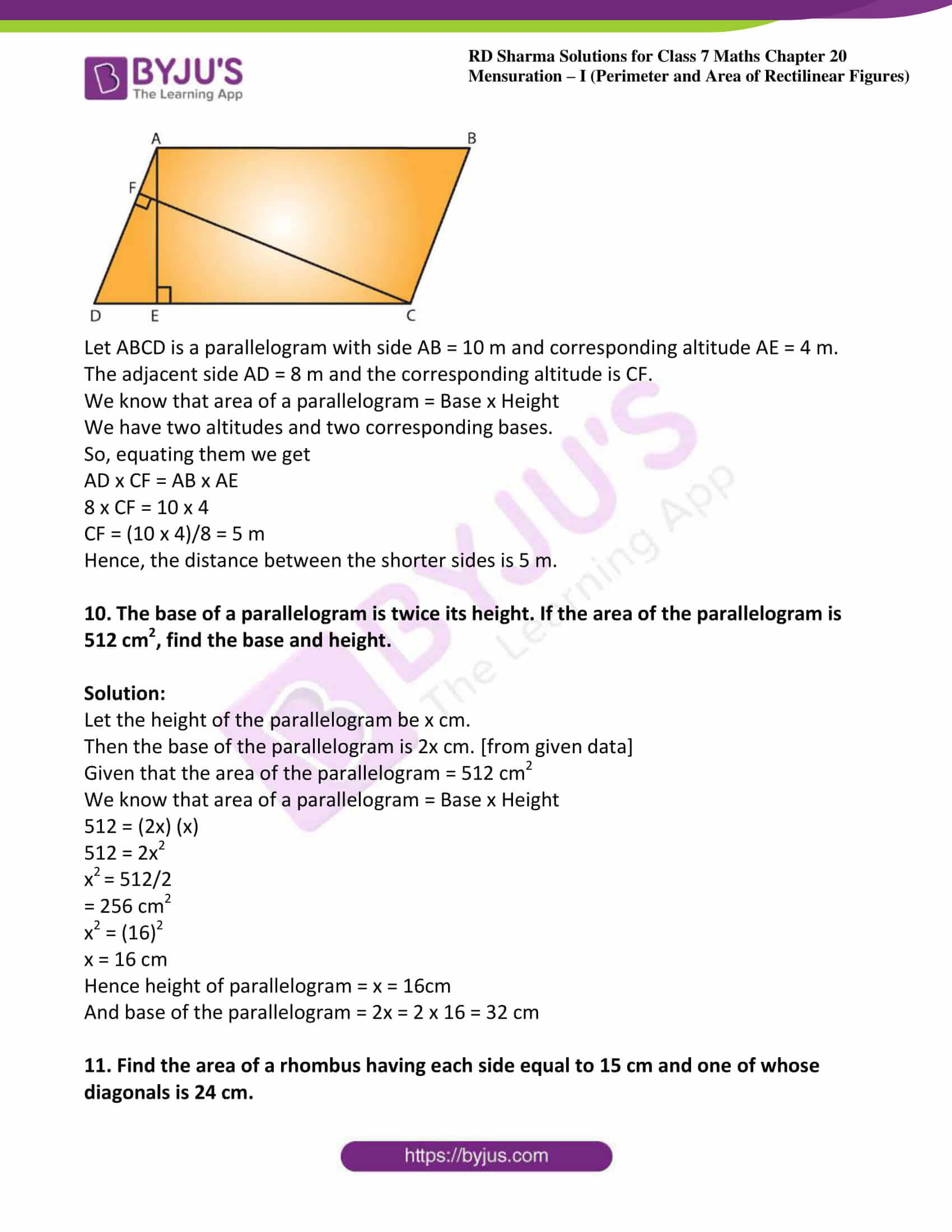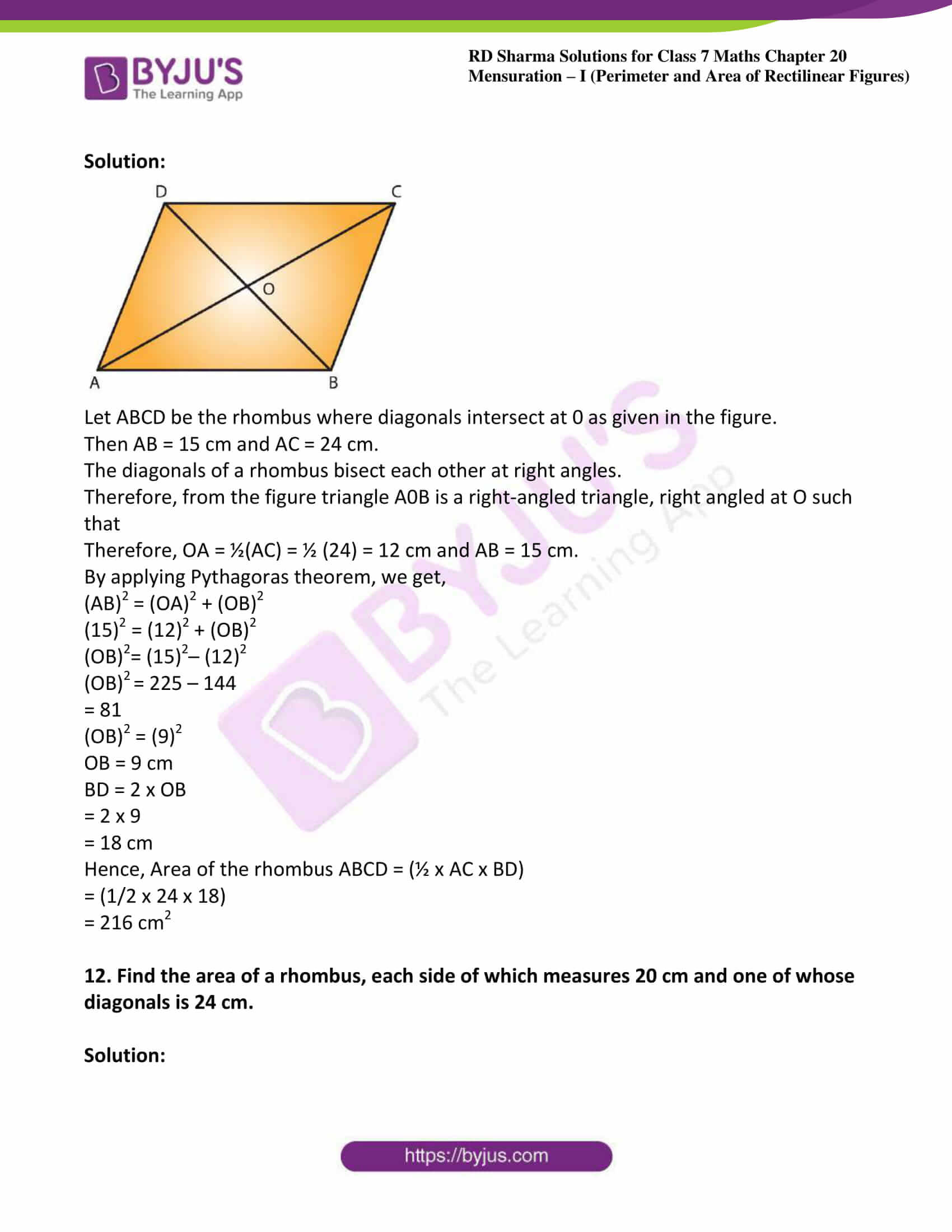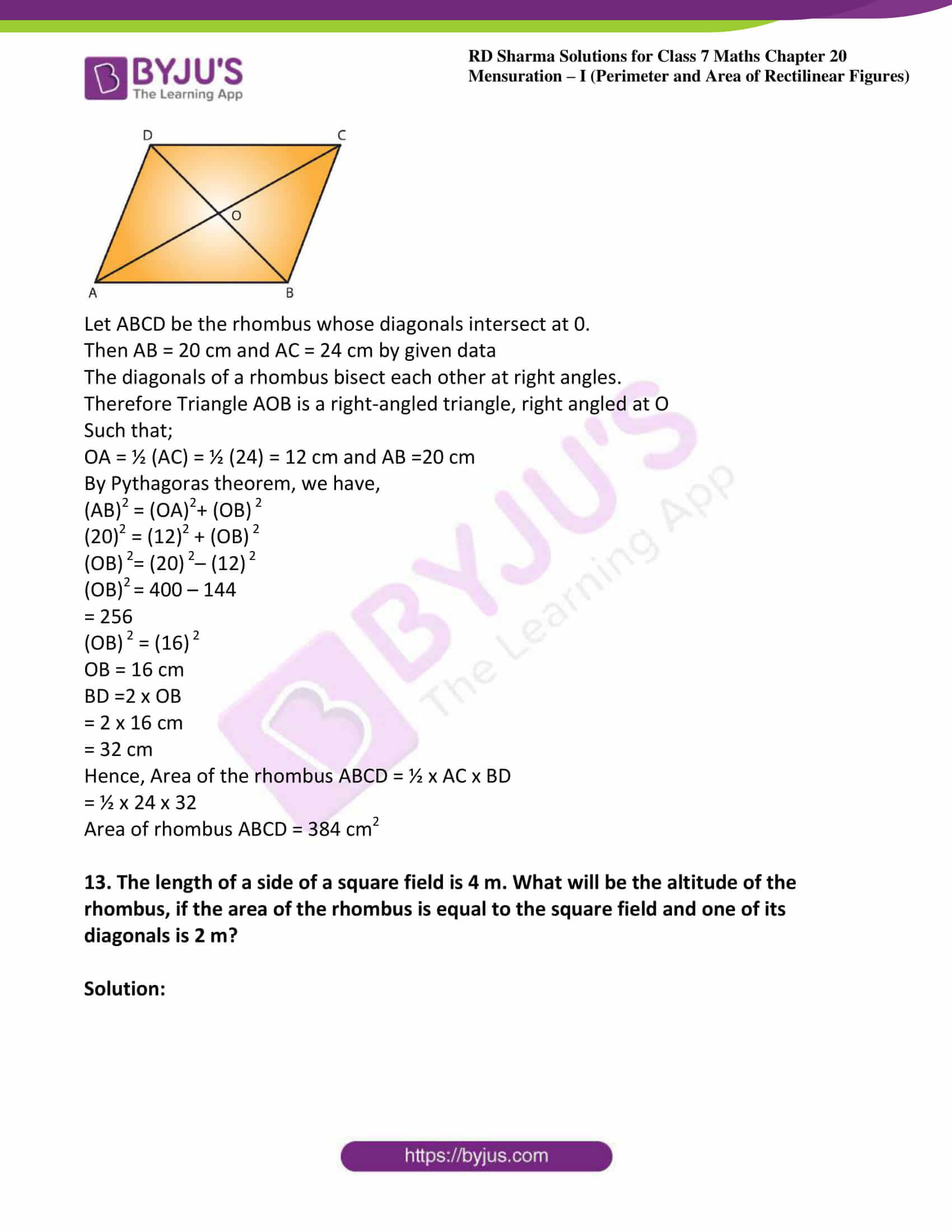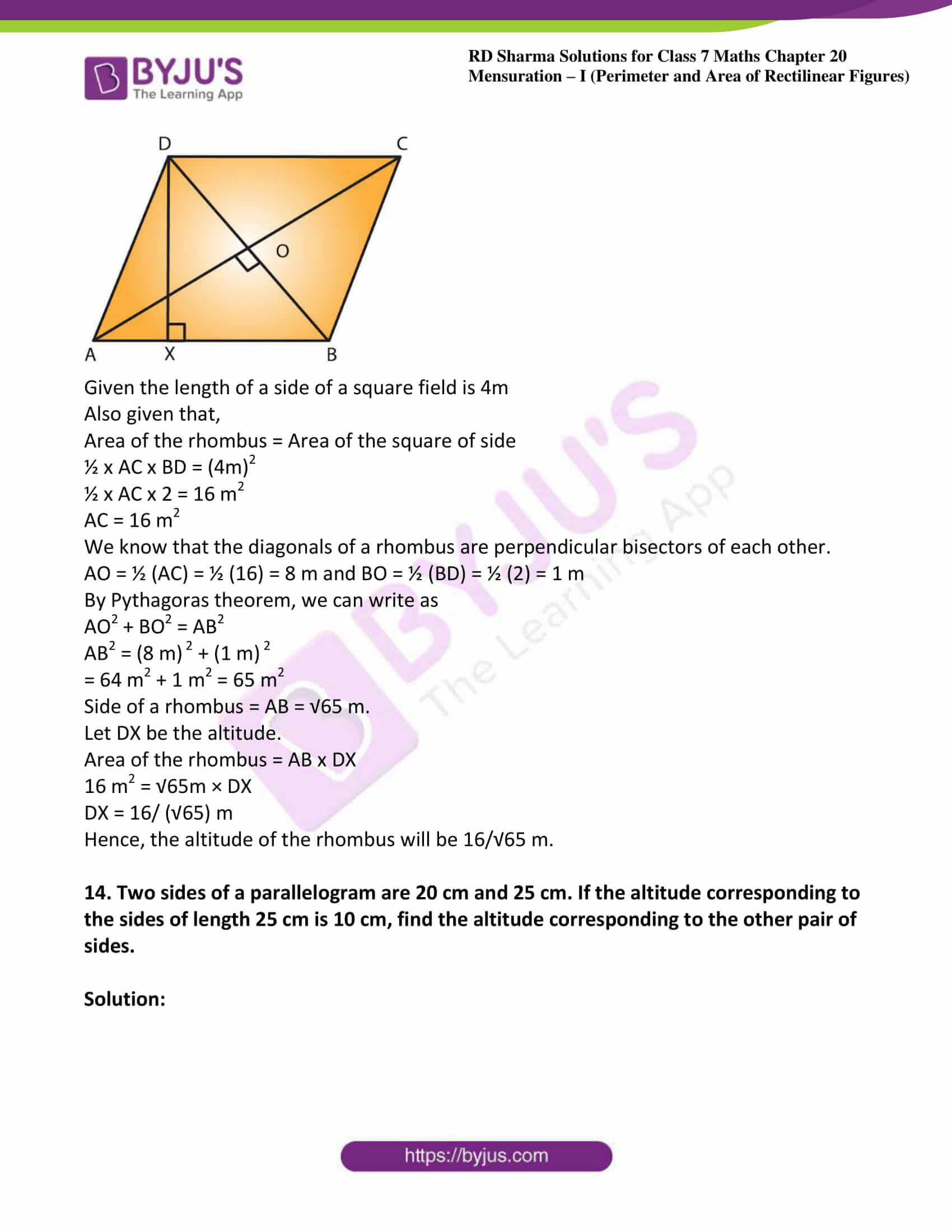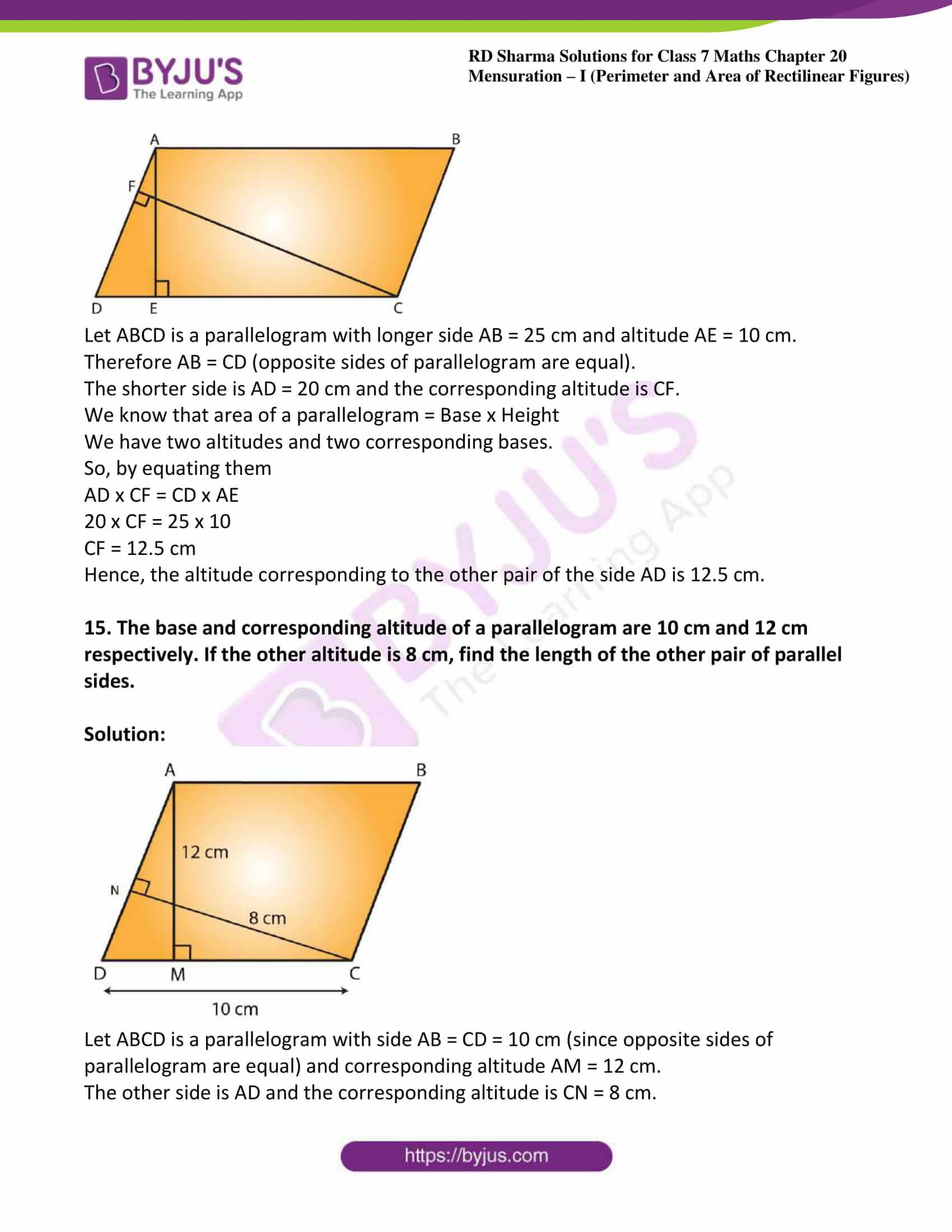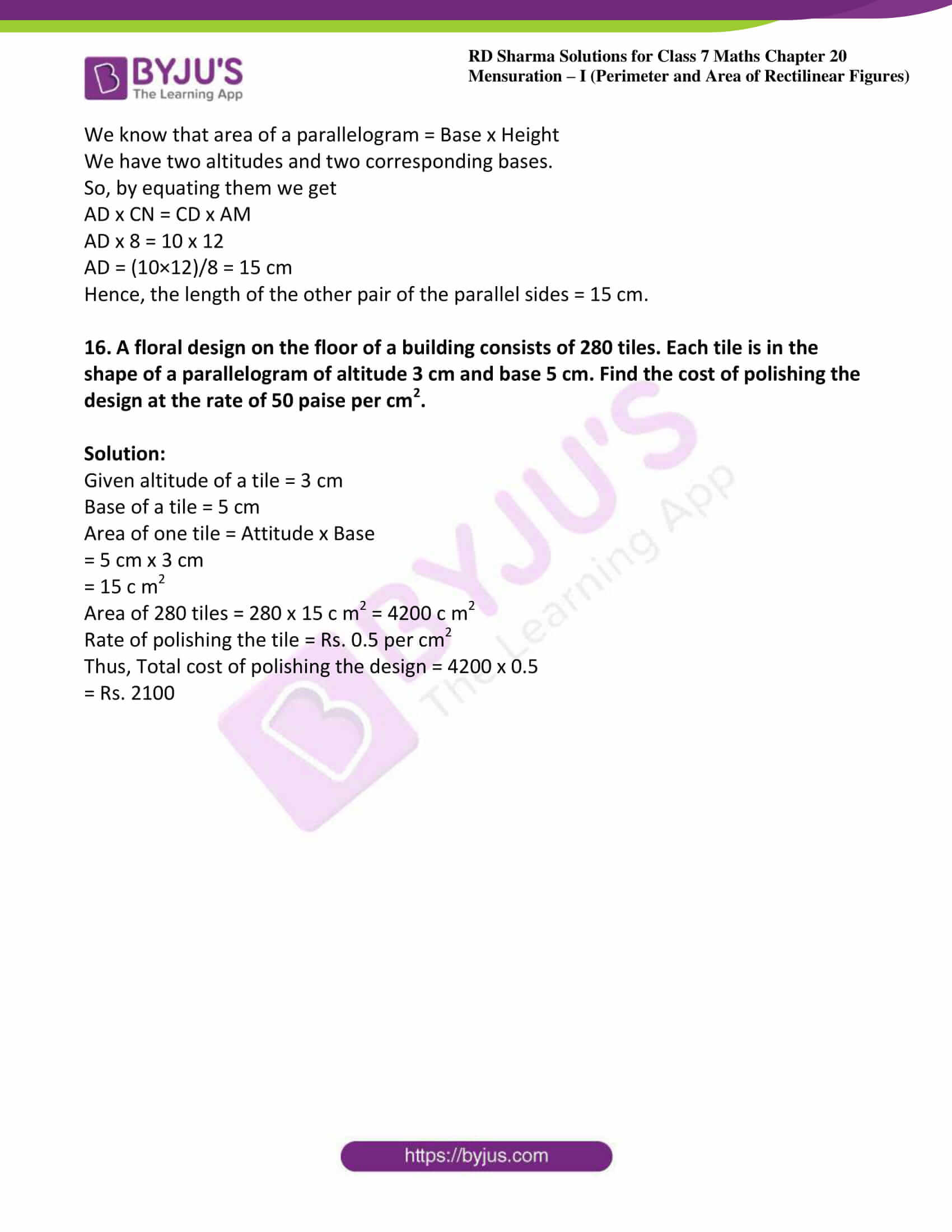### Access answers to Maths RD Sharma Solutions For Class 7 Chapter 20 – Mensuration – I (Perimeter and Area of rectilinear figures) Exercise 20.3

Exercise 20.3 Page No: 20.20

1. Find the area of a parallelogram with base 8 cm and altitude 4.5 cm.

Solution:

Given base = 8 cm and altitude = 4.5 cm

We know that area of the parallelogram = Base x Altitude

= 8 cm x 4.5 cm

Therefore, area of parallelogram = 36 cm2

2. Find the area in square meters of the parallelogram whose base and altitudes are as under

(i) Base =15 dm, altitude = 6.4 dm

(ii) Base =1 m 40 cm, altitude = 60 cm

Solution:

(i) Given base =15 dm, altitude = 6.4 dm

By converting these to standard form we get,

Base = 15 dm = (15 x 10) cm = 150 cm = 1.5 m

Altitude = 6.4 dm = (6.4 x 10) cm = 64 cm = 0.64 m

We know that area of the parallelogram = Base x Altitude

= 1.5 m x 0.64 m

Area of parallelogram = 0.96 m2

(ii) Given base = 1 m 40 cm = 1.4 m [Since 100 cm = 1 m]

Altitude = 60 cm = 0.6 m [Since 100 cm = 1 m]

We know that area of the parallelogram = Base x Altitude

= 1.4 m x 0.6 m

= 0.84 m2

3.  Find the altitude of a parallelogram whose area is 54 d m2 and base is 12 dm.

Solution:

Given area of the given parallelogram = 54 d m2

Base of the given parallelogram = 12 dm

We know that area of the parallelogram = Base x Altitude

Therefore altitude of the given parallelogram = Area/Base

= 54/12 dm

= 4.5 dm

4. The area of a rhombus is 28 m2. If its perimeter be 28 m, find its altitude.

Solution:

Given perimeter of a rhombus = 28 m

But we know that perimeter of a rhombus = 4 (Side)

4(Side) = 28 m

Side = 28/4

Side = 7m

Now, Area of the rhombus = 28 m2

But we know that area of rhombus = Side x Altitude

(Side x Altitude) = 28 m2

(7 x Altitude) = 28 m2

Altitude = 28/7 = 4 m

5. In Fig. 20, ABCD is a parallelogram, DL ⊥ AB and DM ⊥ BC. If AB = 18 cm, BC =12 cm and DM= 9.3 cm, find DL.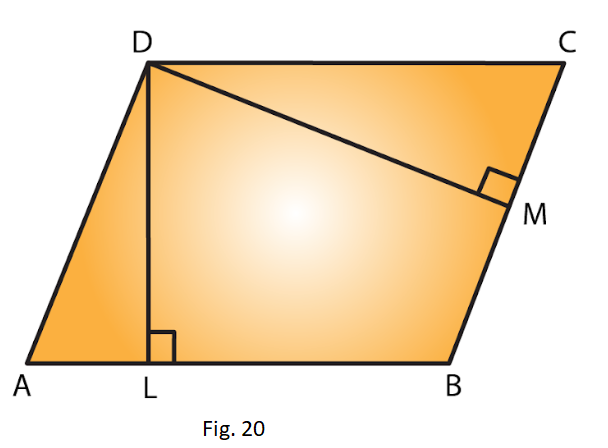Solution:

Given DL ⊥ AB and DM ⊥ BC

Taking BC as the base, BC = 12 cm and altitude DM = 9.3 cm

We know that area of parallelogram ABCD = Base x Altitude

= (12 cm x 9.3 cm)

= 111.6 c m2 ….. Equation (i)

Now, by taking AB as the base,

We have, Area of the parallelogram ABCD = Base x Altitude

= (18 cm x DL) ….. Equation (ii)

From (i) and (ii), we have

18 cm x DL = 111.6 c m2

DL = 111.6/18

= 6.2 cm

6. The longer side of a parallelogram is 54 cm and the corresponding altitude is 16 cm. If the altitude corresponding to the shorter side is 24 cm, find the length of the shorter side.

Solution: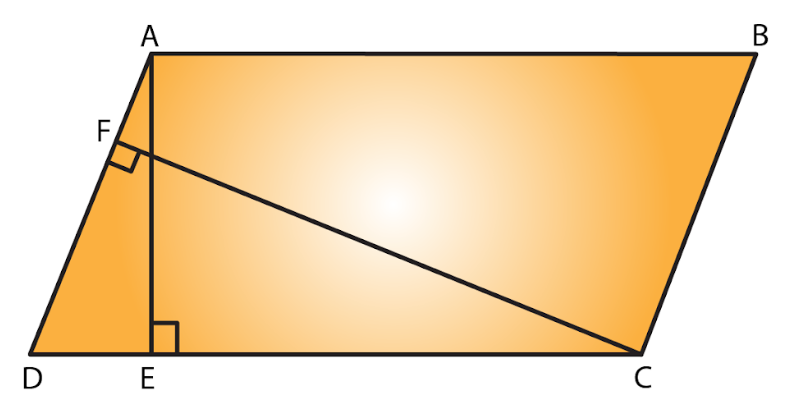Let ABCD is a parallelogram with the longer side AB = 54 cm and corresponding altitude AE = 16 cm.

The shorter side is BC and the corresponding altitude is CF = 24 cm.

We know that area of a parallelogram = base x height.

We have two altitudes and two corresponding bases.

By equating them we get,

½ x BC x CF = ½ x AB x AE

On simplifying, we get

BC x CF = AB x AE

BC x 24 = 54 x 16

BC = (54 × 16)/24

= 36 cm

Hence, the length of the shorter side BC = AD = 36 cm.

7. In Fig. 21, ABCD is a parallelogram, DL ⊥ AB. If AB = 20 cm, AD = 13 cm and area of the parallelogram is 100 c m2, find AL.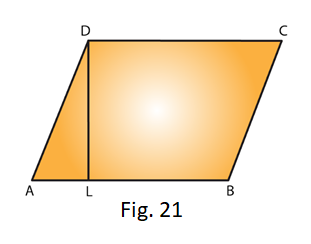Solution:

From the figure we have ABCD is a parallelogram with base AB = 20 cm and corresponding altitude DL.

It is given that the area of the parallelogram ABCD = 100 c m2

We know that the area of a parallelogram = Base x Height

Therefore,

100 = AB x DL

100 = 20 x DL

DL = 100/20 = 5 cm

By observing the picture it is clear that we have to apply the Pythagoras theorem,

Therefore by Pythagoras theorem, we have,

(13)2 = (AL)2+ (5)2

(AL)2 = (13)2 – (5)2

(AL)2 = 169 – 25

= 144

We know that 122 = 144

(AL)2 = (12)2

AL = 12 cm

Hence, length of AL is 12 cm.

8. In Fig. 21, if AB = 35 cm, AD= 20 cm and area of the parallelogram is 560 cm2, find LB.Solution:

From the figure, ABCD is a parallelogram with base AB = 35 cm and corresponding altitude DL.

It is given that the area of the parallelogram ABCD = 560 cm2

Now, Area of the parallelogram = Base x Height

560 cm2 = AB x DL

560 cm2 = 35 cm x DL

DL = 560/35

= 16 cm

Again by Pythagoras theorem, we have, (AD)2 = (AL)2 + (DL)2

(20)2= (AL)2 + (16)2

(AL)= (20)– (16)2

= 400 – 256

= 144

(AL)2 = (12)2

AL = 12 cm

From the figure, AB = AL + LB

35 = 12 + LB

LB = 35 – 12 = 23 cm

Hence, length of LB is 23 cm.

9. The adjacent sides of a parallelogram are 10 m and 8 m. If the distance between the longer sides is 4 m, find the distance between the shorter sides

Solution: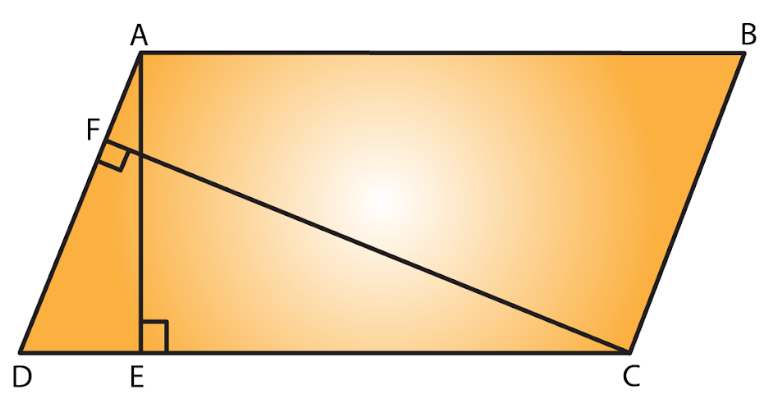Let ABCD is a parallelogram with side AB = 10 m and corresponding altitude AE = 4 m.

The adjacent side AD = 8 m and the corresponding altitude is CF.

We know that area of a parallelogram = Base x Height

We have two altitudes and two corresponding bases.

So, equating them we get

AD x CF = AB x AE

8 x CF = 10 x 4

CF = (10 x 4)/8 = 5 m

Hence, the distance between the shorter sides is 5 m.

10. The base of a parallelogram is twice its height. If the area of the parallelogram is 512 cm2, find the base and height.

Solution:

Let the height of the parallelogram be x cm.

Then the base of the parallelogram is 2x cm. [from given data]

Given that the area of the parallelogram = 512 cm2

We know that area of a parallelogram = Base x Height

512 = (2x) (x)

512 = 2x2

x= 512/2

= 256 cm2

x2 = (16)2

x = 16 cm

Hence height of parallelogram = x = 16cm

And base of the parallelogram = 2x = 2 x 16 = 32 cm

11. Find the area of a rhombus having each side equal to 15 cm and one of whose diagonals is 24 cm.

Solution: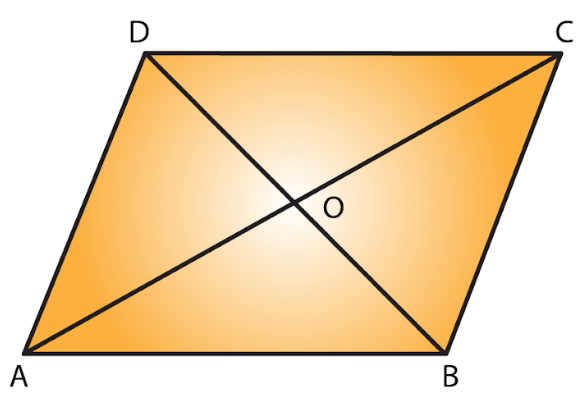Let ABCD be the rhombus where diagonals intersect at 0 as given in the figure.

Then AB = 15 cm and AC = 24 cm.

The diagonals of a rhombus bisect each other at right angles.

Therefore, from the figure triangle A0B is a right-angled triangle, right angled at O such that

Therefore, OA = ½(AC) = ½ (24) = 12 cm and AB = 15 cm.

By applying Pythagoras theorem, we get,

(AB)2 = (OA)2 + (OB)2

(15)2 = (12)2 + (OB)2

(OB)2= (15)2– (12)2

(OB)= 225 – 144

= 81

(OB)2 = (9)2

OB = 9 cm

BD = 2 x OB

= 2 x 9

= 18 cm

Hence, Area of the rhombus ABCD = (½ x AC x BD)

= (1/2 x 24 x 18)

= 216 cm2

12. Find the area of a rhombus, each side of which measures 20 cm and one of whose diagonals is 24 cm.

Solution:Let ABCD be the rhombus whose diagonals intersect at 0.

Then AB = 20 cm and AC = 24 cm by given data

The diagonals of a rhombus bisect each other at right angles.

Therefore Triangle AOB is a right-angled triangle, right angled at O

Such that;

OA = ½ (AC) = ½ (24) = 12 cm and AB =20 cm

By Pythagoras theorem, we have,

(AB)2 = (OA)2+ (OB) 2

(20)2 = (12)2 + (OB) 2

(OB) 2= (20) 2– (12) 2

(OB)= 400 – 144

= 256

(OB) 2 = (16) 2

OB = 16 cm

BD =2 x OB

= 2 x 16 cm

= 32 cm

Hence, Area of the rhombus ABCD = ½ x AC x BD

= ½ x 24 x 32

Area of rhombus ABCD = 384 cm2

13. The length of a side of a square field is 4 m. What will be the altitude of the rhombus, if the area of the rhombus is equal to the square field and one of its diagonals is 2 m?

Solution: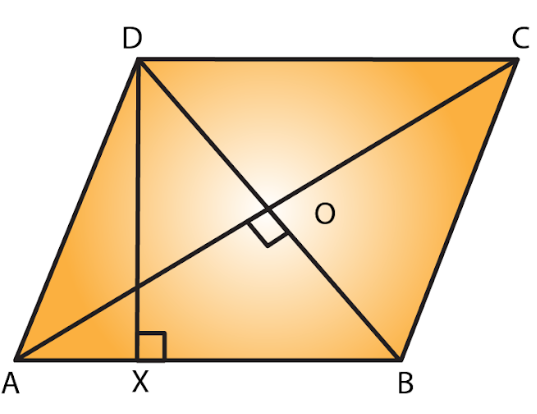Given the length of a side of a square field is 4m

Also given that,

Area of the rhombus = Area of the square of side

½ x AC x BD = (4m)2

½ x AC x 2 = 16 m2

AC = 16 m2

We know that the diagonals of a rhombus are perpendicular bisectors of each other.

AO = ½ (AC) = ½ (16) = 8 m and BO = ½ (BD) = ½ (2) = 1 m

By Pythagoras theorem, we can write as

AO2 + BO2 = AB2

AB2 = (8 m) 2 + (1 m) 2

= 64 m2 + 1 m2 = 65 m2

Side of a rhombus = AB = √65 m.

Let DX be the altitude.

Area of the rhombus = AB x DX

16 m2 = √65m × DX

DX = 16/ (√65) m

Hence, the altitude of the rhombus will be 16/√65 m.

14. Two sides of a parallelogram are 20 cm and 25 cm. If the altitude corresponding to the sides of length 25 cm is 10 cm, find the altitude corresponding to the other pair of sides.

Solution: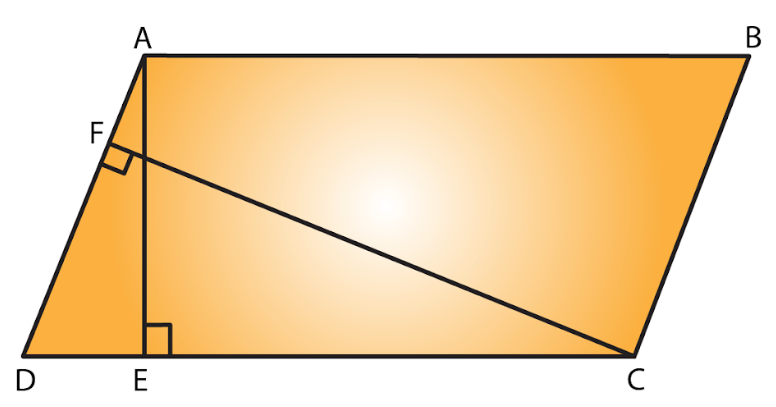Let ABCD is a parallelogram with longer side AB = 25 cm and altitude AE = 10 cm.

Therefore AB = CD (opposite sides of parallelogram are equal).

The shorter side is AD = 20 cm and the corresponding altitude is CF.

We know that area of a parallelogram = Base x Height

We have two altitudes and two corresponding bases.

So, by equating them

AD x CF = CD x AE

20 x CF = 25 x 10

CF = 12.5 cm

Hence, the altitude corresponding to the other pair of the side AD is 12.5 cm.

15. The base and corresponding altitude of a parallelogram are 10 cm and 12 cm respectively. If the other altitude is 8 cm, find the length of the other pair of parallel sides.

Solution: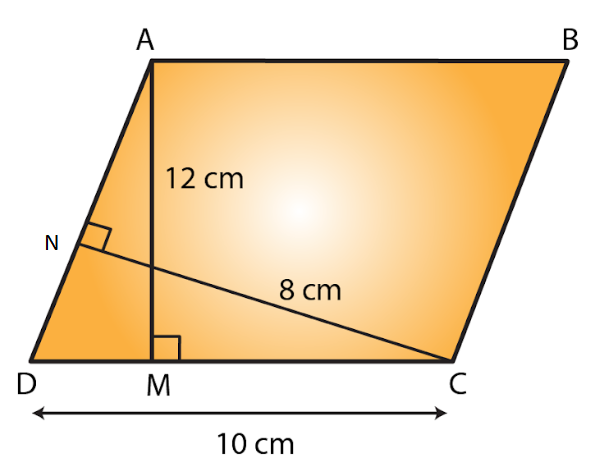Let ABCD is a parallelogram with side AB = CD = 10 cm (since opposite sides of parallelogram are equal) and corresponding altitude AM = 12 cm.

The other side is AD and the corresponding altitude is CN = 8 cm.

We know that area of a parallelogram = Base x Height

We have two altitudes and two corresponding bases.

So, by equating them we get

AD x CN = CD x AM

AD x 8 = 10 x 12

AD = (10×12)/8 = 15 cm

Hence, the length of the other pair of the parallel sides = 15 cm.

16. A floral design on the floor of a building consists of 280 tiles. Each tile is in the shape of a parallelogram of altitude 3 cm and base 5 cm. Find the cost of polishing the design at the rate of 50 paise per cm2.

Solution:

Given altitude of a tile = 3 cm

Base of a tile = 5 cm

Area of one tile = Attitude x Base

= 5 cm x 3 cm

= 15 c m2

Area of 280 tiles = 280 x 15 c m2 = 4200 c m2

Rate of polishing the tile = Rs. 0.5 per cm2

Thus, Total cost of polishing the design = 4200 x 0.5

= Rs. 2100